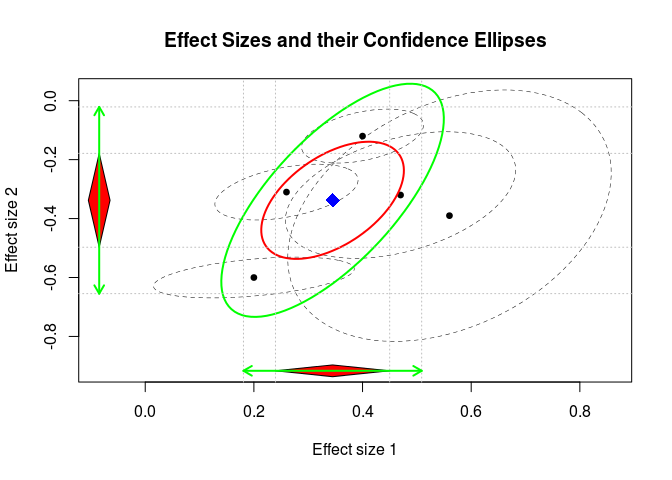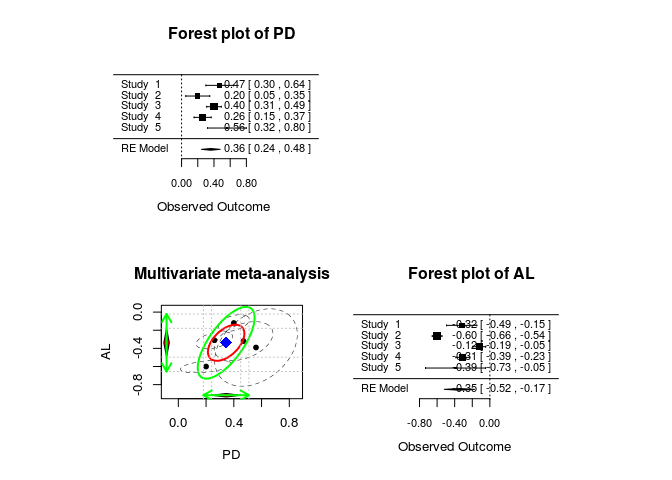# Introduction

• The metaSEM package provides functions to conducting univariate and multivariate meta-analysis using a structural equation modeling approach via the OpenMx package. It also implemented the two-stage structural equation modeling (TSSEM) approach to conducting fixed- and random-effects meta-analytic structural equation modeling (MASEM) on correlation/covariance matrices.

• The metaSEM package is based on the following papers:
• Cheung, M.W.-L. (2008). A model for integrating fixed-, random-, and mixed-effects meta-analyses into structural equation modeling. Psychological Methods, 13, 182-202.
• Cheung, M.W.-L. (2009). Constructing approximate confidence intervals for parameters with structural equation models. Structural Equation Modeling, 16, 267–294.
• Cheung, M.W.-L. (2010). Fixed-effects meta-analyses as multiple-group structural equation models. Structural Equation Modeling, 17, 481-509.
• Cheung, M.W.-L. (2013). Implementing restricted maximum likelihood estimation in structural equation models. Structural Equation Modeling, 20, 157-167.
• Cheung, M.W.-L. (2013). Multivariate meta-analysis as structural equation models. Structural Equation Modeling, 20, 429-454.
• Cheung, M.W.-L. (2014). MetaSEM: an R package for meta-analysis using structural equation modeling. Frontiers in Psychology, 5 (1521).
• Cheung, M.W.-L. (2014). Fixed- and random-effects meta-analytic structural equation modeling: Examples and analyses in R. Behavior Research Methods, 46, 29-40.
• Cheung, M.W.-L. (2014). Modeling dependent effect sizes with three-level meta-analyses: A structural equation modeling approach. Psychological Methods, 19, 211-229.
• Cheung, M.W.-L. (2015). Meta-Analysis: A Structural Equation Modeling Approach. Wiley.
• Cheung, M.W.-L., & Chan, W. (2004). Testing dependent correlation coefficients via structural equation modeling. Organizational Research Methods, 7, 206–223.
• Cheung, M.W.-L., & Chan, W. (2005). Meta-analytic structural equation modeling: A two-stage approach. Psychological Methods, 10, 40-64.
• Cheung, M.W.-L., & Chan, W. (2009). A two-stage approach to synthesizing covariance matrices in meta-analytic structural equation modeling. Structural Equation Modeling, 6, 28-53.

# Examples

## Univariate meta-analysis

### Random-effects model

## Load the library
library(metaSEM)

## Show the first few studies of the data set
head(Becker83)
  study    di   vi percentage items
1     1 -0.33 0.03         25     2
2     2  0.07 0.03         25     2
3     3 -0.30 0.02         50     2
4     4  0.35 0.02        100    38
5     5  0.69 0.07        100    30
6     6  0.81 0.22        100    45
## Random-effects meta-analysis with ML
summary( meta(y=di, v=vi, data=Becker83) )

Call:
meta(y = di, v = vi, data = Becker83)

95% confidence intervals: z statistic approximation
Coefficients:
Estimate Std.Error    lbound    ubound z value Pr(>|z|)
Intercept1  0.174734  0.113378 -0.047482  0.396950  1.5412   0.1233
Tau2_1_1    0.077376  0.054108 -0.028674  0.183426  1.4300   0.1527

Q statistic on the homogeneity of effect sizes: 30.64949
Degrees of freedom of the Q statistic: 9
P value of the Q statistic: 0.0003399239

Heterogeneity indices (based on the estimated Tau2):
Estimate
Intercept1: I2 (Q statistic)   0.6718

Number of studies (or clusters): 10
Number of observed statistics: 10
Number of estimated parameters: 2
Degrees of freedom: 8
-2 log likelihood: 7.928307
OpenMx status1: 0 ("0" or "1": The optimization is considered fine.
Other values may indicate problems.)

### Fixed-effects model

## Fixed-effects meta-analysis by fixiing the heterogeneity variance at 0
summary( meta(y=di, v=vi, data=Becker83, RE.constraints=0) )

Call:
meta(y = di, v = vi, data = Becker83, RE.constraints = 0)

95% confidence intervals: z statistic approximation
Coefficients:
Estimate Std.Error    lbound    ubound z value Pr(>|z|)
Intercept1  0.100640  0.060510 -0.017957  0.219237  1.6632  0.09627 .
---
Signif. codes:  0 '***' 0.001 '**' 0.01 '*' 0.05 '.' 0.1 ' ' 1

Q statistic on the homogeneity of effect sizes: 30.64949
Degrees of freedom of the Q statistic: 9
P value of the Q statistic: 0.0003399239

Heterogeneity indices (based on the estimated Tau2):
Estimate
Intercept1: I2 (Q statistic)        0

Number of studies (or clusters): 10
Number of observed statistics: 10
Number of estimated parameters: 1
Degrees of freedom: 9
-2 log likelihood: 17.86043
OpenMx status1: 0 ("0" or "1": The optimization is considered fine.
Other values may indicate problems.)

### Mixed-effects model

## Mixed-effects meta-analysis with "log(items)" as the predictor
summary( meta(y=di, v=vi, x=log(items), data=Becker83) ) 

Call:
meta(y = di, v = vi, x = log(items), data = Becker83)

95% confidence intervals: z statistic approximation
Coefficients:
Estimate   Std.Error      lbound      ubound z value
Intercept1 -3.2015e-01  1.0981e-01 -5.3539e-01 -1.0492e-01 -2.9154
Slope1_1    2.1088e-01  4.5084e-02  1.2251e-01  2.9924e-01  4.6774
Tau2_1_1    1.0000e-10  2.0095e-02 -3.9386e-02  3.9386e-02  0.0000
Pr(>|z|)
Intercept1  0.003552 **
Slope1_1   2.905e-06 ***
Tau2_1_1    1.000000
---
Signif. codes:  0 '***' 0.001 '**' 0.01 '*' 0.05 '.' 0.1 ' ' 1

Q statistic on the homogeneity of effect sizes: 30.64949
Degrees of freedom of the Q statistic: 9
P value of the Q statistic: 0.0003399239

Explained variances (R2):
y1
Tau2 (no predictor)    0.0774
Tau2 (with predictors) 0.0000
R2                     1.0000

Number of studies (or clusters): 10
Number of observed statistics: 10
Number of estimated parameters: 3
Degrees of freedom: 7
-2 log likelihood: -4.208024
OpenMx status1: 0 ("0" or "1": The optimization is considered fine.
Other values may indicate problems.)

## Multivariate meta-analysis

### Random-effects model

## Show the data set
Berkey98
  trial pub_year no_of_patients   PD    AL var_PD cov_PD_AL var_AL
1     1     1983             14 0.47 -0.32 0.0075    0.0030 0.0077
2     2     1982             15 0.20 -0.60 0.0057    0.0009 0.0008
3     3     1979             78 0.40 -0.12 0.0021    0.0007 0.0014
4     4     1987             89 0.26 -0.31 0.0029    0.0009 0.0015
5     5     1988             16 0.56 -0.39 0.0148    0.0072 0.0304
## Multivariate meta-analysis with a random-effects model
mult1 <- meta(y=cbind(PD, AL), v=cbind(var_PD, cov_PD_AL, var_AL), data=Berkey98)

summary(mult1)

Call:
meta(y = cbind(PD, AL), v = cbind(var_PD, cov_PD_AL, var_AL),
data = Berkey98)

95% confidence intervals: z statistic approximation
Coefficients:
Estimate  Std.Error     lbound     ubound z value  Pr(>|z|)
Intercept1  0.3448392  0.0536312  0.2397239  0.4499544  6.4298 1.278e-10
Intercept2 -0.3379381  0.0812480 -0.4971812 -0.1786951 -4.1593 3.192e-05
Tau2_1_1    0.0070020  0.0090497 -0.0107351  0.0247391  0.7737    0.4391
Tau2_2_1    0.0094607  0.0099698 -0.0100797  0.0290010  0.9489    0.3427
Tau2_2_2    0.0261445  0.0177409 -0.0086270  0.0609161  1.4737    0.1406

Intercept1 ***
Intercept2 ***
Tau2_1_1
Tau2_2_1
Tau2_2_2
---
Signif. codes:  0 '***' 0.001 '**' 0.01 '*' 0.05 '.' 0.1 ' ' 1

Q statistic on the homogeneity of effect sizes: 128.2267
Degrees of freedom of the Q statistic: 8
P value of the Q statistic: 0

Heterogeneity indices (based on the estimated Tau2):
Estimate
Intercept1: I2 (Q statistic)   0.6021
Intercept2: I2 (Q statistic)   0.9250

Number of studies (or clusters): 5
Number of observed statistics: 10
Number of estimated parameters: 5
Degrees of freedom: 5
-2 log likelihood: -11.68131
OpenMx status1: 0 ("0" or "1": The optimization is considered fine.
Other values may indicate problems.)
## Plot the effect sizes
plot(mult1)## Plot the effect sizes with the forest plots
## Load the library for forest plots
library("metafor")

## Create extra panels for the forest plots
plot(mult1, diag.panel=TRUE, main="Multivariate meta-analysis",
axis.label=c("PD", "AL"))

## Forest plot for PD
forest( rma(yi=PD, vi=var_PD, data=Berkey98) )
title("Forest plot of PD")

## Forest plot for AL
forest( rma(yi=AL, vi=var_AL, data=Berkey98) )
title("Forest plot of AL")### Fixed-effects model

## Fixed-effects meta-analysis by fixiing the heterogeneity variance component at
## a 2x2 matrix of 0.
summary( meta(y=cbind(PD, AL), v=cbind(var_PD, cov_PD_AL, var_AL), data=Berkey98,
RE.constraints=matrix(0, nrow=2, ncol=2)) )

Call:
meta(y = cbind(PD, AL), v = cbind(var_PD, cov_PD_AL, var_AL),
data = Berkey98, RE.constraints = matrix(0, nrow = 2, ncol = 2))

95% confidence intervals: z statistic approximation
Coefficients:
Estimate Std.Error    lbound    ubound z value  Pr(>|z|)
Intercept1  0.307219  0.028575  0.251212  0.363225  10.751 < 2.2e-16 ***
Intercept2 -0.394377  0.018649 -0.430929 -0.357825 -21.147 < 2.2e-16 ***
---
Signif. codes:  0 '***' 0.001 '**' 0.01 '*' 0.05 '.' 0.1 ' ' 1

Q statistic on the homogeneity of effect sizes: 128.2267
Degrees of freedom of the Q statistic: 8
P value of the Q statistic: 0

Heterogeneity indices (based on the estimated Tau2):
Estimate
Intercept1: I2 (Q statistic)        0
Intercept2: I2 (Q statistic)        0

Number of studies (or clusters): 5
Number of observed statistics: 10
Number of estimated parameters: 2
Degrees of freedom: 8
-2 log likelihood: 90.88326
OpenMx status1: 0 ("0" or "1": The optimization is considered fine.
Other values may indicate problems.)

### Mixed-effects model

## Multivariate meta-analysis with "publication year-1979" as a predictor
summary( meta(y=cbind(PD, AL), v=cbind(var_PD, cov_PD_AL, var_AL), data=Berkey98,
x=scale(pub_year, center=1979)) )

Call:
meta(y = cbind(PD, AL), v = cbind(var_PD, cov_PD_AL, var_AL),
x = scale(pub_year, center = 1979), data = Berkey98)

95% confidence intervals: z statistic approximation
Coefficients:
Estimate  Std.Error     lbound     ubound z value  Pr(>|z|)
Intercept1  0.3440001  0.0857659  0.1759020  0.5120982  4.0109 6.048e-05
Intercept2 -0.2918175  0.1312797 -0.5491209 -0.0345140 -2.2229   0.02622
Slope1_1    0.0063540  0.1078235 -0.2049762  0.2176842  0.0589   0.95301
Slope2_1   -0.0705888  0.1620966 -0.3882922  0.2471147 -0.4355   0.66322
Tau2_1_1    0.0080405  0.0101206 -0.0117955  0.0278766  0.7945   0.42692
Tau2_2_1    0.0093413  0.0105515 -0.0113392  0.0300218  0.8853   0.37599
Tau2_2_2    0.0250135  0.0170788 -0.0084603  0.0584873  1.4646   0.14303

Intercept1 ***
Intercept2 *
Slope1_1
Slope2_1
Tau2_1_1
Tau2_2_1
Tau2_2_2
---
Signif. codes:  0 '***' 0.001 '**' 0.01 '*' 0.05 '.' 0.1 ' ' 1

Q statistic on the homogeneity of effect sizes: 128.2267
Degrees of freedom of the Q statistic: 8
P value of the Q statistic: 0

Explained variances (R2):
y1     y2
Tau2 (no predictor)    0.0070020 0.0261
Tau2 (with predictors) 0.0080405 0.0250
R2                     0.0000000 0.0433

Number of studies (or clusters): 5
Number of observed statistics: 10
Number of estimated parameters: 7
Degrees of freedom: 3
-2 log likelihood: -12.00859
OpenMx status1: 0 ("0" or "1": The optimization is considered fine.
Other values may indicate problems.)

# Installation and help

• First of most important, you need R to run it. You can install the metaSEM package by running the following command inside an R session:
install.packages("metaSEM")
• The developmental version can be installed from GitHub by running:
library(devtools)
install_github("mikewlcheung/metasem")

## Potential issues with the OpenMx available at CRAN

• The OpenMx available at CRAN includes only the open source SLSQP optimizer.
• If the SLSQP optimizer does not work well for you, e.g., there are many error codes, you may try to rerun the analysis. For example,
random1 <- meta(y=yi, v=vi, data=Hox02)
random1 <- rerun(random1)
summary(random1)
• If you still prefer the non-free NPSOL optimizer, you may install it from the OpenMx website and call it by running:
mxOption(NULL, "Default optimizer", "NPSOL")

## Help

• Reference manual
• Vignette
• If you need help,
• OpenMx discussion forum: A discussion forum for the metaSEM package in OpenMx. You may post technical questions related to metaSEM there. Please include information on the session. It will be helpful if you can include a reproducible example. You may save a copy of your data, say my.df, and attach the content of myData.R in the post by using

sessionInfo()
dump(c("my.df"), file="myData.R")
• Meta-analysis resources: A discussion forum related to the conceptual issues of meta-analytic structural equation modeling.

The files are based on the following versions of R and R packages:

sessionInfo()
R version 3.2.5 (2016-04-14)
Platform: x86_64-pc-linux-gnu (64-bit)
Running under: Ubuntu 14.04.3 LTS

locale:
 LC_CTYPE=en_SG.UTF-8       LC_NUMERIC=C
 LC_TIME=en_SG.UTF-8        LC_COLLATE=en_SG.UTF-8
 LC_MONETARY=en_SG.UTF-8    LC_MESSAGES=en_SG.UTF-8
 LC_PAPER=en_SG.UTF-8       LC_NAME=C
 LC_MEASUREMENT=en_SG.UTF-8 LC_IDENTIFICATION=C

attached base packages:
 parallel  stats     graphics  grDevices utils     datasets  methods
 base

other attached packages:
 metafor_1.9-8   metaSEM_0.9.8   OpenMx_2.5.2    Rcpp_0.12.4
 Matrix_1.2-5    MASS_7.3-45     digest_0.6.9    knitr_1.12.3
 rmarkdown_0.9.5

loaded via a namespace (and not attached):
 lattice_0.20-33 grid_3.2.5      formatR_1.3     magrittr_1.5
 ellipse_0.3-8   evaluate_0.8.3  stringi_1.0-1   tools_3.2.5
 stringr_1.0.0   yaml_2.1.13     htmltools_0.3.5